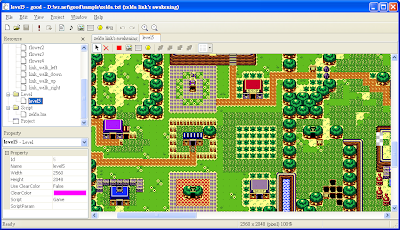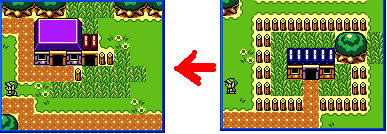## 2009年5月3日 星期日

### 實作迷你薩爾達傳說先以編輯器編輯好遊戲資源。使用地圖TileSet編輯好地圖資源，並開一個新關卡資源加地圖加入。然後再加入幾個簡單的精靈資源，主要是林克的4個行走方向，還有幾朵不同顏色的花。接著就可以開始用Lua來實作Gameplay。

+ + +

+ + +

`Game.OnCreate = function(param) local idLvl = param._id scrolling = false Good.SetPos(idLvl, 320, 1280) KillAndDynaGenObj(idLvl)end`
Game初始化的時候，我們先把關卡的位置捲到小屋前林克所在的小地圖位置，同時初始化用來控制是否作地圖捲動的全域變數scrolling。接著再呼叫函式KillAndDynaGenObj來動態產生小花物件。

KillAndDynaGenObj函式首先會把除了林克以外的所有精靈物件刪除。接著檢查目前地圖可見範圍內的每一塊Tile，假如是小花Tile的話，則在那個地置動態的產生一個小花物件出來(全部共有4種不同顏色的小花)。最後一步則是再把林克物件加入關卡裡，這個動作是重新調整物件的次序，確保林克會畫在其它物件上面。
`function KillAndDynaGenObj(idLvl) local nc = Good.GetChildCount(idLvl) for i = nc,0,-1 do   local idChild = Good.GetChild(idLvl, i)   if (Good.TYPES_OBJ == Good.GetType(idChild) and 14 ~= idChild) then     Good.KillObj(idChild)   end end local x,y = Good.GetPos(idLvl) for i = 0,9 do   for j = 0,7 do     local lx,ly = x + 16 * i, y + 16 * j     local tile = Resource.GetTileByPos(1, lx, ly)     ...(略)   end end Good.AddChild(idLvl, 14)end`
OnStep則檢查scrolling全域變數是否設立來執行捲動，捲動的方向則檢查另一全域變數dir。捲動的時候依據水平或垂直方向決定速度，再配合offset全域變數來控制捲動量。當結束捲動時，再執行一次KillAndDynaGenObj函式重新產生小花物件。
`Game.OnStep = function(param) local idLvl = param._id if (not scrolling) then   return end local x,y = Good.GetPos(idLvl) local spd1, spd2 = 5, 4 if (Input.KEYS_LEFT == dir) then   x = x - spd1   offset = offset + spd1 elseif ...   ....(略) end Good.SetPos(idLvl, x, y) if (Input.KEYS_LEFT == dir or Input.KEYS_RIGHT == dir) then   if (160 <= offset) then     scrolling = false   end elseif (Input.KEYS_DOWN == dir or Input.KEYS_UP == dir) then   if (128 <= offset) then     scrolling = false   end  end  if (not scrolling) then    KillAndDynaGenObj(idLvl)  endend`

+ + +

`Link.OnStep = function(param) local id = param._id local x,y = Good.GetPos(id) if (scrolling) then   local spd1, spd2 = 0.5, 0.5   if (Input.KEYS_LEFT == dir) then     x = x - spd1   elseif ...     ....(略)   end   Good.SetPos(id, x, y)   return endend``Link.OnStep = function(param)  ....(略)  local ox,oy = x,y  local spr  local spd = 0.8  if (Input.IsKeyDown(Input.KEYS_LEFT)) then    x = x - spd    spr = 10  elseif (Input.IsKeyDown(Input.KEYS_RIGHT)) then    x = x + spd    spr = 13  end  if (Input.IsKeyDown(Input.KEYS_DOWN)) then    y = y + spd    spr = 11  elseif (Input.IsKeyDown(Input.KEYS_UP)) then    y = y - spd    spr = 12  end  if (ox == x and oy == y) then    return  end  Good.SetSpriteId(id, spr)end`

`Link.OnStep = function(param)  ....(略)  local cx,cy = x + 8, y + 10  if (Input.IsKeyDown(Input.KEYS_LEFT)) then    cx = x;  elseif (Input.IsKeyDown(Input.KEYS_RIGHT)) then    cx = x + 16  end  if (Input.IsKeyDown(Input.KEYS_DOWN)) then    cy = y + 16  end  if (not IsMoveable(cx, cy)) then    return  endend`

`MoveableTile = {121,122,....(略)}function IsMoveable(x, y)  local tile = Resource.GetTileByPos(1, x, y)  for i,v in ipairs(MoveableTile) do    if (tile == v) then      return true    end  end  return falseend`
IsMoveable原理很簡單，根據指定位置取出地圖Tile，並且和MoveableTile裡所列出的所有可走的Tile作比較作是否可走判定。
`Link.OnStep = function(param)  ....(略)  local idLvl = Good.GetParent(id)  local px,py = Good.GetPos(idLvl)  dir = -1  if (px > x) then    dir = Input.KEYS_LEFT    x = x - 0.5  elseif (px + 160 < x + 16) then    dir = Input.KEYS_RIGHT    x = x + 0.5  end  if (py > y) then    dir = Input.KEYS_UP    y = y - 0.5  elseif (py + 128 < y + 16) then    dir = Input.KEYS_DOWN    y = y + 0.5  end    if (-1 ~= dir) then    offset = 0    scrolling = true  end  Good.SetPos(id, x, y)end`

....(完)

#### 2 則留言:

1.有沒有 linux 版本, 支援 DirectFB ? 或許可以介紹幾個朋友給你, 看能不能 porting 到中華電信 MOD 的 STB 上.

Ramon

2.這是設計成跨平台, 在Windows和iPhone上有Player可以執行, PSP上也有試Port過, 可以執行使用色塊但不包含貼圖的Sample